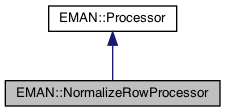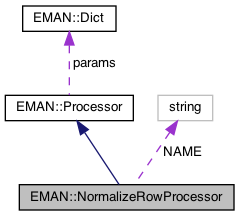EMAN2
EMAN::NormalizeRowProcessor Class Reference

normalizes each row in the image individually More...

`#include <processor.h>`

Inheritance diagram for EMAN::NormalizeRowProcessor:[legend]
Collaboration diagram for EMAN::NormalizeRowProcessor:[legend]

## Public Member Functions

string get_name () const
Get the processor's name. More...

TypeDict get_param_types () const
Get processor parameter information in a dictionary. More...

string get_desc () const
Get the descrition of this specific processor. More...

void process_inplace (EMData *image)
To process an image in-place. More...Public Member Functions inherited from EMAN::Processor
virtual ~Processor ()

virtual EMDataprocess (const EMData *const image)
To proccess an image out-of-place. More...

virtual void process_list_inplace (vector< EMData * > &images)
To process multiple images using the same algorithm. More...

virtual Dict get_params () const
Get the processor parameters in a key/value dictionary. More...

virtual void set_params (const Dict &new_params)
Set the processor parameters using a key/value dictionary. More...

## Static Public Member Functions

static ProcessorNEW ()Static Public Member Functions inherited from EMAN::Processor
static string get_group_desc ()
Get the description of this group of processors. More...

static void EMFourierFilterInPlace (EMData *fimage, Dict params)
Compute a Fourier-filter processed image in place. More...

static EMDataEMFourierFilter (EMData *fimage, Dict params)
Compute a Fourier-processor processed image without altering the original image. More...

## Static Public Attributes

static const string NAME = "normalize.rows"Public Types inherited from EMAN::Processor
enum  fourier_filter_types {
TOP_HAT_LOW_PASS , TOP_HAT_HIGH_PASS , TOP_HAT_BAND_PASS , TOP_HOMOMORPHIC ,
GAUSS_LOW_PASS , GAUSS_HIGH_PASS , GAUSS_BAND_PASS , GAUSS_INVERSE ,
GAUSS_HOMOMORPHIC , BUTTERWORTH_LOW_PASS , BUTTERWORTH_HIGH_PASS , BUTTERWORTH_HOMOMORPHIC ,
KAISER_I0 , KAISER_SINH , KAISER_I0_INVERSE , KAISER_SINH_INVERSE ,
SHIFT , TANH_LOW_PASS , TANH_HIGH_PASS , TANH_HOMOMORPHIC ,
}
Fourier filter Processor type enum. More...Protected Attributes inherited from EMAN::Processor
Dict params

## Detailed Description

normalizes each row in the image individually

Definition at line 6176 of file processor.h.

## ◆ get_desc()

 string EMAN::NormalizeRowProcessor::get_desc ( ) const
inlinevirtual

Get the descrition of this specific processor.

This function must be overwritten by a subclass.

Returns
The description of this processor.

Implements EMAN::Processor.

Definition at line 6196 of file processor.h.

6197 {
6198 return "Modifies each row in the image individually. Default behavior is to divide each value by the mean value of the row, as long as the mean>0.";
6199 }

## ◆ get_name()

 string EMAN::NormalizeRowProcessor::get_name ( ) const
inlinevirtual

Get the processor's name.

Each processor is identified by a unique name.

Returns
The processor's name.

Implements EMAN::Processor.

Definition at line 6179 of file processor.h.

6180 {
6181 return NAME;
6182 }
static const string NAME
Definition: processor.h:6201

References NAME.

## ◆ get_param_types()

 TypeDict EMAN::NormalizeRowProcessor::get_param_types ( ) const
inlinevirtual

Get processor parameter information in a dictionary.

Each parameter has one record in the dictionary. Each record contains its name, data-type, and description.

Returns
A dictionary containing the parameter info.

Reimplemented from EMAN::Processor.

Definition at line 6189 of file processor.h.

6190 {
6191 TypeDict d;
6192 d.put("unitlen",EMObject::BOOL, "Adjusts the length of the 'row vector' to be 1.0 without adjusting the mean");
6193 return d;
6194 }
TypeDict is a dictionary to store <string, EMObject::ObjectType> pair.
Definition: emobject.h:305
void put(const string &key, EMObject::ObjectType o, const string &desc="")
Definition: emobject.h:330

References EMAN::EMObject::BOOL, and EMAN::TypeDict::put().

## ◆ NEW()

 static Processor * EMAN::NormalizeRowProcessor::NEW ( )
inlinestatic

Definition at line 6184 of file processor.h.

6185 {
6186 return new NormalizeRowProcessor();
6187 }
normalizes each row in the image individually
Definition: processor.h:6177

## ◆ process_inplace()

 void NormalizeRowProcessor::process_inplace ( EMData * image )
virtual

To process an image in-place.

For those processors which can only be processed out-of-place, override this function to just print out some error message to remind user call the out-of-place version.

Parameters
 image The image to be processed.

Implements EMAN::Processor.

Definition at line 4926 of file processor.cpp.

4927{
4928 if (!image) {
4929 LOGWARN("NULL Image");
4930 return;
4931 }
4932
4933
4934
4935
4936 float *rdata = image->get_data();
4937 int nx = image->get_xsize();
4938 int ny = image->get_ysize();
4939 int nz = image->get_zsize();
4940
4941 if (nz > 1) {
4942 // Muyuan 08/2018: normalize by slice when nz>1
4943 // since this processor is not used anyways..
4944 for (int z = 0; z < nz; z++) {
4945 double row_sum = 0;
4946 double row_sqr = 0;
4947 for (int y = 0; y < ny; y++) {
4948 for (int x = 0; x < nx; x++) {
4949 float d = rdata[x + y * nx + z*nx*ny];
4950 row_sum += d;
4951 d*=d;
4952 row_sqr += d;
4953 }
4954 }
4955 double row_mean = row_sum / nx / ny;
4956 double row_std = row_sqr / nx / ny;
4957 row_std = sqrt(row_std - row_mean*row_mean);
4958 for (int y = 0; y < ny; y++) {
4959 for (int x = 0; x < nx; x++) {
4960 rdata[x + y * nx+ z*nx*ny] -= (float)row_mean;
4961 rdata[x + y * nx+ z*nx*ny] /= (float)row_std;
4962 }
4963 }
4964
4965 }
4966
4967
4968// LOGERR("row normalize only works for 2D image");
4969// return;
4970 }
4971
4972 else {
4973 if (params.has_key("unitlen")) {
4974 for (int y = 0; y < ny; y++) {
4975 double row_len = 0;
4976 for (int x = 0; x < nx; x++) {
4977 row_len += pow((double)rdata[x + y * nx],2.0);
4978 }
4979 row_len=sqrt(row_len);
4980 if (row_len==0) row_len=1.0;
4981
4982 for (int x = 0; x < nx; x++) {
4983 rdata[x + y * nx] /= (float)row_len;
4984 }
4985 }
4986 image->update();
4987 return;
4988 }
4989
4990 for (int y = 0; y < ny; y++) {
4991 double row_sum = 0;
4992 for (int x = 0; x < nx; x++) {
4993 row_sum += rdata[x + y * nx];
4994 }
4995
4996 double row_mean = row_sum / nx;
4997 if (row_mean <= 0) {
4998 row_mean = 1;
4999 }
5000
5001 for (int x = 0; x < nx; x++) {
5002 rdata[x + y * nx] /= (float)row_mean;
5003 }
5004 }
5005 }
5006 image->update();
5007}
#define rdata(i)
Definition: analyzer.cpp:592
bool has_key(const string &key) const
Ask the Dictionary if it as a particular key.
Definition: emobject.h:511
EMData * sqrt() const
return square root of current image
#define LOGWARN
Definition: log.h:53
#define y(i, j)
Definition: projector.cpp:1516
#define x(i)
Definition: projector.cpp:1517

References EMAN::Dict::has_key(), LOGWARN, EMAN::Processor::params, rdata, sqrt(), x, and y.

## ◆ NAME

 const string NormalizeRowProcessor::NAME = "normalize.rows"
static

Definition at line 6201 of file processor.h.

Referenced by get_name().

The documentation for this class was generated from the following files: